# KSEEB SSLC Class 10 Science Solutions Chapter 13 Magnetic Effects of Electric Current

KSEEB SSLC Class 10 Science Solutions Chapter 13 Magnetic Effects of Electric Current are part of KSEEB SSLC Class 10 Science Solutions. Here we have given Karnataka SSLC Class 10 Science Solutions Chapter 13 Magnetic Effects of Electric Current.

## Karnataka SSLC Class 10 Science Solutions Chapter 13 Magnetic Effects of Electric Current

### KSEEB SSLC Class 10 Science Chapter 13 Intext Questions

Text Book Part I Page No. 118

Question 1.
Why does a compass needle get deflected when brought near a bar magnet?
A compass needle get deflected when brought near a bar magnet because a compass needle is in fact, a small bar magnet. The ends of the compass needle point approximately towards north and south directions.

Text Book Part I Page No. 122

Question 2.
Draw magnetic Held lines around a bar magnet.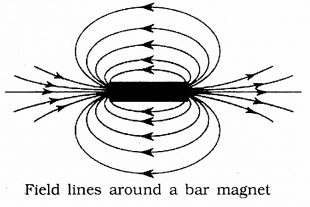Field lines around a bar magnet

Question 3.
List the properties of magnetic field lines.
The properties are:

• They travel from north pole to south pole outside the magnet and south pole to north pole inside the magnet.
• They are closed and continuous curves.
• Two magnetic field lines never intersect each other. If the lines intersect, then at the point of intersection there would be two directions [the needle would point towards two directions] for the same magnetic fields which is not possible.
• The number of field lines per unit area is the measure of the strength of magnetic field, which is maximum at poles. The magnetic field is strong, where the field lines are close together and weak where the lines are far apart.

Question 4.
Why don’t two magnetic field lines intersect each other?
Two magnetic fields lines of force never intersect each other. If the lines intersect, then at [the point of intersection there would be two directions [the needle would point towards two directions] for the same magnetic field, which is not possible.

Text Book Part I Page No. 123, 124

Question 1.
Consider a circular loop of wire lying in the plane of the table. Let the current pass through the loop clockwise. Apply the right- hand rule to find out the direction of the magnetic field inside and outside the loop.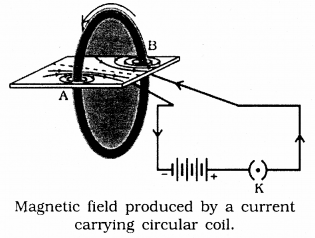Since the current passes through the loop in a clockwise direction, therefore the front face of the loop will be the south pole and the back face, ie, the face touching the table will be north pole. According to right-hand rule, the direction of the magnetic field inside the loop will be pointing downward. Outside the loop, the direction of the magnetic field will be upward.

Question 2.
The magnetic field in a given region is uniform. Draw a diagram to represent it.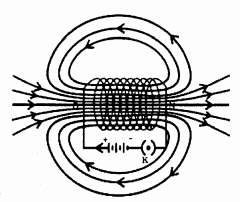The figure indicates that the magnetic field is the same at all points in the solenoid. That is field is uniform inside the solenoid.

Question 3.
Choose the correct option.
The magnetic field inside a long straight solenoid-carrying current
(a) is zero.
(b) decreases as we move towards its end.
(c) increases as we move towards its end.
(d) is the same at all points.
(d) is the same at all points.

Text Book Part I Page No. 125, 126

Question 1.
Which of the following property of a proton can change while it moves freely in a magnetic field? (There may be more than one correct answer.)
(a) mass
(b) speed
(c) velocity
(d) momentum
(c) velocity
(d) momentum.

Question 2.
In Activity 13.7, how do we think the displacement of rod AB will be affected if

1. current in rod AB is increased;
2. a stronger horse-shoe magnet is used; and
3. length of the rod AB is increased?

1. displacement of A is increased.
2. If a stronger horse-shoe magnet is used magnetic field is increasing.
3. current flows is more.

Question 3.
A positively-charged particle (alpha-particle) projected towards west is deflected towards north by a magnetic field. The direction of magnetic field is
(a) towards south
(b) towards east
(c) downward
(d) upward
(d) upward.
Since the positively charged particle alpha particle projected towards west, so the direction of current is towards west. Now the deflection is towards north, so the force is towards north. Now hold the forefinger, centre finger and thumb of our left – hand at right angles to one another. Let us adjust the hand in such a way that our centre finger points towards west and thumb points towards north. If we look at our forefinger, it will be pointing, upward. Thus, the magnetic field is in the upward direction. So, the correct answer is (d).

Text Book Part I Page No 127

Question 1.
State Fleming’s left-hand rule.
According to this rule, stretch the thumb, forefinger, and middle finger of your left hand such that they are mutually perpendicular. If the first finger points in the direction of the Magnetic field and the second finger in the direction of current, then the thumb will point in the direction of motion or the force acting on the conductor.

Question 2.
What is the principle of an electric motor?
A current-carrying conductor when placed in a magnetic field experiences a force. This is the principle of an electric motor.

Question 3.
What is the role of the split ring in an electric motor?
The split ring reverse the direction of current in the armature coil after every half rotation i.e., it acts as a commutator. The reversal of current reverses, the direction of the forces acting on the two arms of the armature after every half rotation. This allows the armature coil to rotate continuously in the same direction.

Text Book Part I Page No. 130

Question 1.
Explain different ways to induce current in a coil.
We can induce current in a coil either by moving it in a magnetic field or by changing the magnetic around it. It is convenient in most situations to move the coil in a magnetic field.

Text Book Part I Page No. 131

Question 1.
State the principle of an electric generator.
Based on the phenomenon of electromagnetic induction, electric generator are prepared. In an electric generator, Mechanical energy is used to rotate a conductor in a magnetic field to produce electricity. This is the principle of an electric generator.

Question 2.
Name some sources of direct current.
Dry cell, Battery and D.C. generator.

Question 3.
Which sources produce alternating current?
A.C. generator and D.C. generator.

Question 4.
Choose the correct option. A rectangular coil of copper wires is rotated in a magnetic field. The direction of the induced current changes once in each
(a) two revolutions
(b) one revolution
(c) half revolution
(d) one-fourth revolution.
(c) half revolution.

Text Book Part I Page No. 132

Question 1.
Name two safety measures commonly used in electric circuits and appliances.

1. Electric fuse
2. Earthing wire.

Question 2.
An electric oven of 2 kW power rating is operated in a domestic electric circuit (220 V) that has a current rating of 5 A. What result do you expect? Explain.
P = VI
Here P = 2 KW = 2000 W
V = 220The current drawn by this electric oven is 9 A whereas the fuse in the circuit is ( only 5 A capacity. When a high current of 9 A flows through the 5 A fuse, the fuse wire will get heated too much, melt and break, the circuit. Therefore, when a 2 kW power rating electric oven is operated in a circuit having a 5 A fuse will blow off cutting off the power supply in this circuit.

Question 3.
What precaution should be taken to avoid the overloading of domestic electric circuits?

1. Each appliance has a separate switch to ON/OFF the flow of current through it.
2. The use of an electric fuse prevents the electric circuit and the appliance from possible damage by stopping the flow of unduly high electric current.
3. We should not connect too many appliances to a single socket to prevent overloading.

### KSEEB SSLC Class 10 Science Chapter 13 Textbook Exercises

Question 1.
Which of the following correctly describes the magnetic Held near a long straight wire?
(a) The field consists of straight lines perpendicular to the wire.
(b) The field consists of straight lines parallel to the wire.
(c) The field consists of radial lines originating from the wire.
(d) The field consists of concentric circles centred on the wire.
(d) The field consists of concentric circles centred on the wire.

Question 2.
The phenomenon of electro-magnetic induction is
(a) the process of charging a body.
(b) the process of generating magnetic field due to a current passing through a coil.
(c) producing induced current in a coil due to relative motion between a magnet and the coil.
(d) the process of rotating a coil of an electric motor.
(c) producing induced current in a coil due to relative motion between a magnet and the coil.

Question 3.
The device used for producing electric current is called a
(a) generator
(b) galvanometer
(c) ammeter
(d) motor.
(a) generator.

Question 4.
The essential difference between an AC generator and a DC generator is that
(a) AC generator has an electro-magnet while a DC generator has permanent magnet.
(b) DC generator will generate a higher voltage.
(c) AC generator will generate a higher voltage.
(d) AC generator has slip rings while the DC generator has a commutator.
(c) AC generator will generate a higher voltage.

Question 5.
At the time of short circuit, the current in the circuit
(a) reduces substantially.
(b) does not change.
(c) increases heavily.
(d) vary continuously.
(c) increases heavily.

Question 6.
State whether the following statements are true or false.
(a) An electric motor converts mechanical energy into electrical energy.
(b) An electric generator works on the principle of electromagnetic induction.
(c) The field at the centre of a long circular coil carrying current will be parallel straight lines.
(d) A wire with a green insulation is usually the live wire of an electric supply.
(a) False
(b) true
(c) true
(d) False.

Question 7.
List two methods of producing magnetic fields.

1. Permanent magnet
2. Electromagnet.

Question 8.
How does a solenoid behave like a magnet? Can you determine the north and south poles of a current-carrying solenoid with the help of a bar magnet? Explain.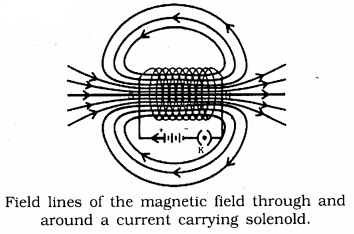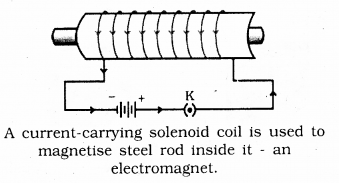One end of the solenoid behaves as a magnetic north pole, while the other behaves as the south pole. The field lines inside the solenoid are in the form of parallel straight lines. This indicates that the magnetic field is the same at the points inside the solenoid.

As shown in figure a strong magnetic field produced inside a solenoid can be used to magnetise a piece of Magnetic material, like soft iron, when placed inside the coil. The magnet so formed is called an electromagnet.

Question 9.
When is the force experienced by a current-carrying conductor placed in a magnetic field largest?
If the direction of magnetic field and flow of electric current are mutually perpendicular then force experienced by a current-carrying conductor in a magnetic field is largest.

Question 10.
Imagine that you are sitting in a chamber with your back to one wall. An electron beam, moving horizontally from back wall towards the front wall, is deflected by a strong magnetic field to your right side. What is the direction of magnetic field?
According to Fleming’s left-hand rule, the magnetic field acts in the vertically downward direction.
Note that the direction of current will be opposite to that of the electron beam.

Question 11.
Draw a labelled diagram of an electric motor. Explain its principle and working. What is the function of a split ring in an electric motor?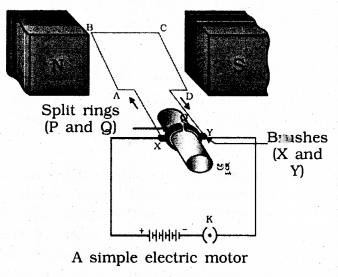Principle:
An electric motor is a rotating device that converts electrical energy to mechanical energy working.

Working:
Current in the coil ABCD enters from the source battery through conducting brush X and flow back to the battery through brush Y. Notice that the current in the Arm AB of the coil flows from A to B. In arm CD it flows from C to D that is opposite to the direction of current through arm AB on applying Fleming’s left hand rule for the direction of force on a current¬carrying conductor in a magnetic field. We find that the force acting on arm AB pushes it downwards while the force acting on arm CD pushes it upwards.

Thus the coil and the Axle O, mounted free to turn about an axis, rotate anti-clockwise at half rotation. Q makes contact with the brush X and P with brush Y. Therefore the current in the coil gets reversed and flows along the path DCBA. The reversal of current also reverses the direction of force acting on the two arms AB and CD. Thus the arm AB of the coil that was earlier pushed down, is now pushed up and the arm CD previously pushed up is pushed down. There is a continuous rotation of the coil and to the axle.
Split rings in electric motors acts as a commutator.

Question 12.
Name some devices in which electric motors are used.
Electric motor is used as an important component in electric fans, refrigerators, mixers, washing machines, computers, MP3 players etc.

Question 13.
A coil of insulated copper wire is connected to a galvanometer. What will happen if a bar magnet is

1. pushed into the coil
2. withdrawn from inside the coil
3. held stationary inside the coil?

1. There is a momentary deflection in the needle of the galvanometer.
2. Now the galvanometer is deflected towards the left showing that the current is now set up in the direction opposite to the first.
3. When the coil is kept stationary with respect to the magnet, the deflection of the galvanometer drops to zero.

Question 14.
Two circular coils A and B are placed closed to each other. If the current in the coil A is changed, will some current be induced in coil B? Give reason.
If the current in the coil A is changed there is a change in its magnetic field. By this electricity is induced in B. This is called Electromagnetic induction.

Question 15.
State the rule to determine the direction of a

1. magnetic field produced around a straight conductor carrying current,
2. force experienced by a current-carrying straight conductor placed in a magnetic field which is perpendicular to it, and
3. current induced in a coil due to its rotation in a magnetic field.

(i) Right-hand thumb rule: If the current-carrying conductor is held in the right hand such that the thumb points in the direction of the current, then the direction of the curl of the fingers will be given the direction of the magnetic field.

(ii) Fleming’slefthandrule: Stretch the forefinger, the central finger of the right hand mutually perpendicular to each other. If the forefinger points in the direction of the magnetic field, the central finger in the direction of the current, then the thumb points in the direction of a force in the conductor.

(iii) Fleming’s right-hand rule: Stretch the thumb/ forefinger and the central finger of the right hand mutually perpendicular to each other. If the forefinger points in the direction of the magnetic field, thumb in the direction conductor, then the central finger points in the direction of current induced in the conductor.

Question 16.
Explain the underlying principle and working of an electric generator by drawing a labelled diagram. What is the function of the brushes?Principle: In an electric generator, mechanical energy is used to rotate a conductor in a magnetic field to produce electricity. It is working on the principle of electromagnetic induction.

Working: When the Axle attached to the two rings is rotated such that the arm AB moves up (and the arm CD moves down) in the magnetic field produced by the permanent magnet. Let us say the coil ABCD is rotated clockwise in the arrangement. By applying Fleming’s right-hand rule, the induced currents are set up in these arms along with the directions AB and CD. Thus an induced current flows in the direction ABCD. If there are a larger number of turns in the coil, the current generated in each turn adds up to give a large current through the coil. This means that the current in the external circuit flows from B2 and B1.

After half a rotation, arm CD starts moving up and AB moving down. As a result, the directions of the induced currents in both the arms change, giving rise to the net induced current in the direction DCBA. The current in the external circuit now flows from B1 to B2. Thus after every half rotation the polarity of the current in the respective arms changes.

There are two brushes and in the electric generator, one brush is at all times in contact with the arm moving up in the field, while the other is in contact with the arm moving down. Because of these Brushes unidirectional current is produced.

Question 17.
When does an electric short circuit occur?
Overloading can occur when the live wire and the neutral wire come into direct current (This occurs when the insulation of wires is damaged or there is a fault in the appliance) In such a situation, the current in the circuit abruptly increases. This is called short-circuiting.

Question 18.
What is the function of an earth wire? Why is it necessary to earth metallic appliances?
This is used as a safety measure, especially for those appliances that have a metallic body, for example, electric press, toaster, table fan, refrigerator, etc. The metallic body is connected to the earth wire which provides a low-resistance conducting path for the current. Thus earth wire ensures that any leakage of current to the metallic body of the appliance keeps its potential to that of the earth and the user may not get a severe electric shock.

### KSEEB SSLC Class 10 Science Chapter 13 Additional Questions and Answers

1. Fill in the blanks:

Question 1.
Magnetic field is a quantity that has both …….. and ……
Magnitude, direction.

Question 2.
An electric current through a metallic conductor produces a ……. around it.
Magnetic field.

Question 3.
In electric motors, the …… acts as a commutator
split ring.

Question 4.
…… current reverses its direction periodically.